franciscop/ola

🌊 Smooth animation library for interpolating numbers
Latest commit a113aff May 21, 2019
Type Name Latest commit message Commit time
Failed to load latest commit information..github May 14, 2019docs May 13, 2019.gitignore May 12, 2019LICENSE May 10, 2019ola.js May 17, 2019ola.min.js May 17, 2019ola.test.js May 17, 2019package.json May 17, 2019readme.md May 20, 2019

OlaSmooth animation library for inbetweening / interpolating numbers in realtime: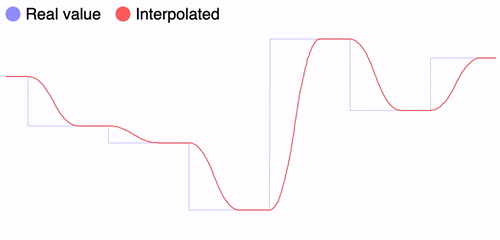```// Start tracking the value
const pos = Ola({ y: 0 });

// Set the value to update async
pos.set({ y: 100 });

// Read the evolution over time
setInterval(() => graph(pos.y), 5);```

It works with multiple values/dimensions: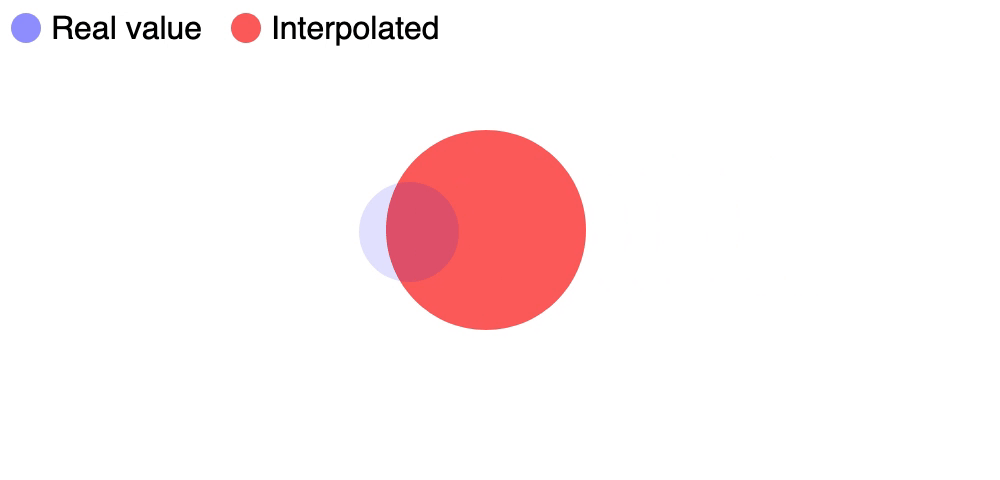```const pos = Ola({ x: 0, y: 0 });

pos.set({ x: e.pageX, y: e.pageY });
});

setInterval(() => {
ball.style.left = `\${pos.x}px`;
ball.style.top = `\${pos.y}px`;
}, 10);```

Also works great with many instances since they are independent: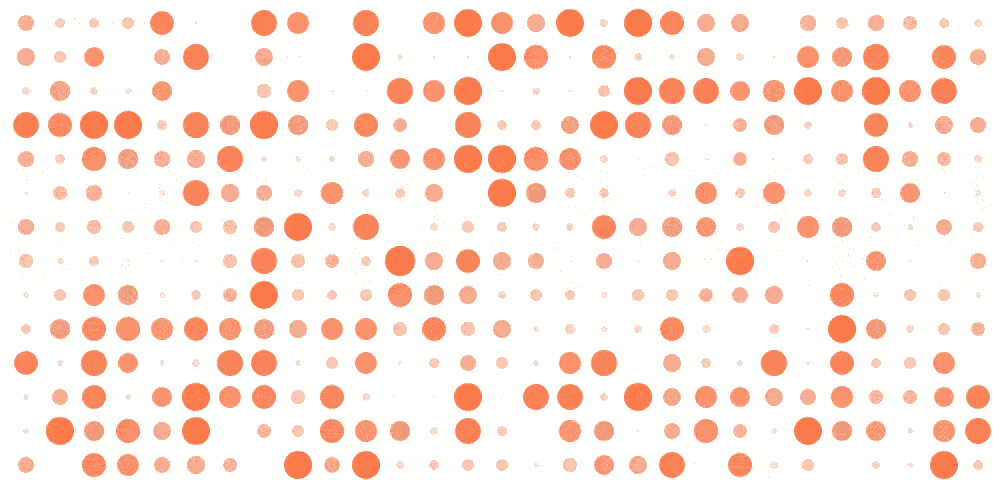```// Generates 1000 instances seamlessly
const dots = Ola(Array(1000).fill(0));

setInterval(() => dots.forEach((dot, i) => {
dots[i] = Math.random();
}), 600);

// ... read + paint screen here```

Tip: click on the GIFs for a live demo with the code :)

Getting started

Install it with npm:

``````npm install ola
``````

Then import it and use it:

```import Ola from "ola";
const pos = Ola({ x: 0 });
console.log(pos.x); // 0```

If you prefer to use a CDN:

```<script src="https://cdn.jsdelivr.net/npm/ola"></script>
<script type="text/javascript">
const pos = Ola({ x: 0 });
console.log(pos.x); // 0
</script>```

Documentation

There are three distinct operations that can be run: creating an instance, setting it to update and reading it.

Create an instance

`Ola(initial, time = 300);`

The first parameter is the initial value. It can be either a single number, or an object of `key:numbers` or an array of numbers:

```const heater = Ola(20); // Alias of `{ value: 20 }`
const motor = Ola({ angle: 180 }); // A named parameter for clarity
const position = Ola({ x: 0, y: 0 }); // Any number of properties
const heights = Ola([0, 0, 0, 0]); // A group of heights```

The second parameter is how long the transition will last. It should be a number that represents the time in milliseconds:

```const heater = Ola(20); // Default = 300 ms
const motor = Ola({ angle: 180 }, 1000); // Turn the motor slowly
const position = Ola({ x: 0, y: 0 }, 100); // Quick movements for the position
const heights = Ola([0, 0, 0, 0], 300); // 300, same as the default```

Passing a single number as a parameter is the same as passing `{ value: num }`, we are just helping by setting a shortname. It is offered for convenience, but recommend not mixing both styles in the same project.

It works with Javascript numbers, but please keep things reasonable (under `Number.MAX_VALUE / 10`):

```console.log(Ola(100));
console.log(Ola(-100));
console.log(Ola(0.001));
console.log(Ola(1 / 100));```

The time it takes to update can also be updated while setting the value, which will update it for any subsequent transition:

```// All `pos.set()` will take 1 full second
const pos = Ola({ x: 0 }, 1000);
pos.set({ x: 100 }, 3000);```

Update the value

```heater.value = 25; // Since the constructor used a number, use `.value`
motor.angle = 90; // Turn -90 degrees from before
position.set({ x: 100, y: 100 }); // Move 0,0 => 100,100
heights = 120; // Move the second (0-index) item to 120```

When we update a property it is not updated instantaneously (that's the whole point of this library), but instead it's set to update asynchronously:

```const pos = Ola({ x: 0 });
pos.set({ x: 100 });

// 0 - still hasn't updated
console.log(pos.x);

// 100 - after 300ms it's fully updated
setTimeout(() => console.log(pos.x), 1000);```

Remember that if you set the value as `Ola(10)`, this is really an alias for `Ola({ value: 10 })`, so use the property `.value` to update it:

```heater.value = 25;
heater.set({ value: 25 });```

You can see in this graph, the blue line is the value that is set though `.set()`, while the red line is the value that reading it returns:```log(heater.value); // Since the constructor used a number, use `.value`
log(motor.angle); // Read as an object property
log(position.get("x")); // Find the X value
log(heights); // Move the first item to 120```

You can read the value at any time, and the value will be calculated at that moment in time:

```const pos = Ola({ x: 0 });
pos.set({ x: 100 });

setInterval(() => {
// It will update every time it's read
console.log(pos.x);
}, 10);```

In contrast to other libraries, there's no need to tick/update the function every N ms or before reading the value, since `Ola()` uses math functions you should just read it when needed.

Features

While there are some other great libraries like Tween, this one has some improvements:

Smooth in realtime

Other libraries don't move smoothly when there's an update while the previous transition is still ongoing. Ola makes sure there are no harsh corners: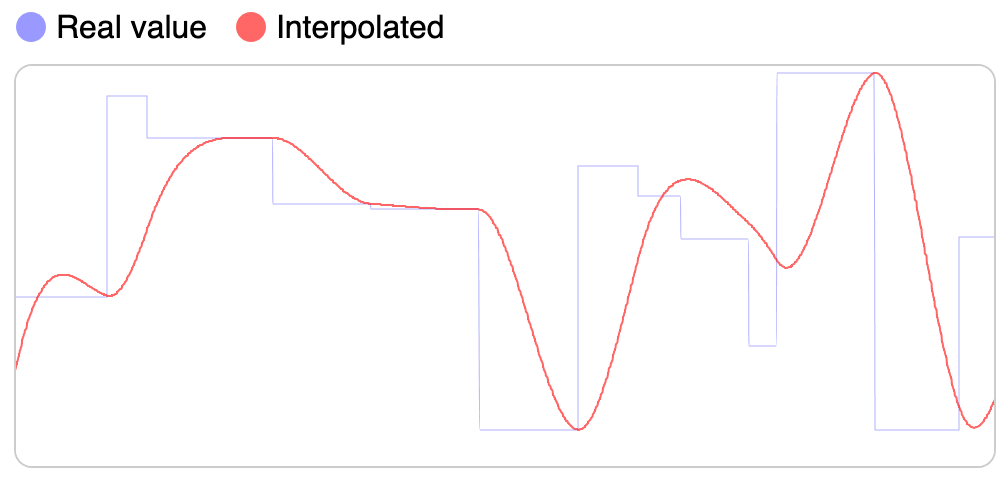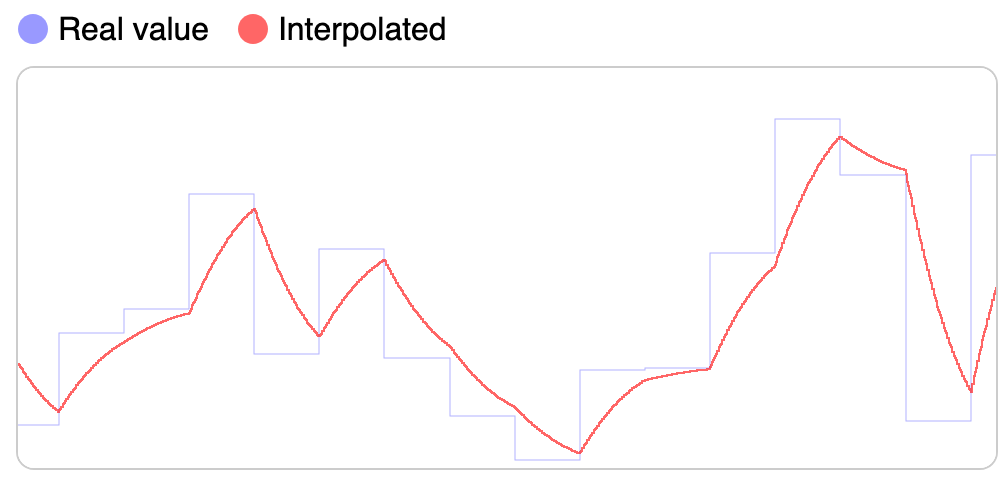Smooth interpolation with Ola() Harsh interpolation with Tweenmax

Status of libraries updating animation mid-way:

Since this is driven by mathematical equations, the library doesn't calculate any value until it needs to be read/updated. It will also only change the one we need instead of all of the values:

```const position = Ola({ x: 0, y: 0 });
position.x = 10; // Only updates X
console.log(position.x); // Calculates only X position, not y```

Not only this is great for performance, but it also makes for a clean self-contained API where each instance is independent and portable.

Others from Author

Like this project? Francisco has many more! Check them out:

• server.js - a batteries-included Node.js server
• translate.js - to easily translate text on the browser and Node.js
You can’t perform that action at this time.